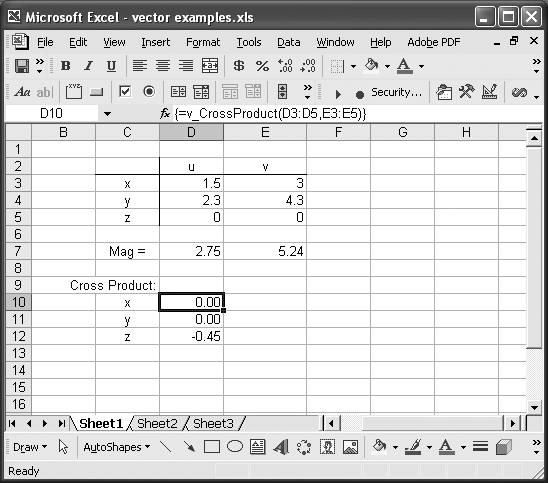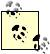# Building Support for Vectors

Problem

You want to perform vector-based calculations , like vector cross and dot products, but can't find any vector functions in Excel.

Solution

Excel does not provide any built-in functions to perform vector calculations; however, you can add your own custom functions without too much effort.

Discussion

Since VBA allows you to write custom functions that can then be called directly from your Excel spreadsheet, you can easily add functions to perform vector calculations.

Figure 7-4 shows an example spreadsheet containing two vectors, u and v, whose x-, y-, and z-components are stored in separate cells.

Figure 7-4. Vector exampleCells D7 and E7 show the magnitudes of these vectors. These magnitudes are computed using a custom function called v_Mag. The syntax for v_Mag is =v_Mag(cell range), where cell range is a cell reference consisting of three cells containing the vector components, x, y, and z. Example 7-1 shows the VBA code for v_Mag. (See Recipe 2.2 for more information on writing VBA functions.)

Example 7-1. v_Mag

 ```Public Function v_Mag(v As Range) As Double Dim x As Double Dim y As Double Dim z As Double   x = v.Cells(1).Value y = v.Cells(2).Value z = v.Cells(3).Value   v_Mag = Sqr(x ^ 2 + y ^ 2 + z ^ 2) End Function ```

The only parameter for this function is a cell range, v, that represents the selected cell range containing the three vector components, x, y, and z. The first three lines of this function declare three local variables in which to store the vector components. You really don't need to use local variables in this example, but I did so to make the remainder of the code more readable.

The next three lines set the values of the local variables to the values contained in the input cell range. Since v is of type Range, you can access its Cells property to retrieve the value for each cell. Each cell in the range is referred to using a 1-based array index as shown.

Finally, the last line of this function computes the magnitude of the vector and returns the result as a Double type scalar value.The VBA function for square root is Sqr, whereas the Excel function for square root is SQRT. This is another example of inconsistencies between VBA and Excel function names.

Computing the vector cross product is a little more involved than simply computing the magnitude of a vector. For the cross product, we need to return the three components of the resulting vector as an array in Excel. This means we need to write a VBA function that returns an array instead of a scalar. The resulting function will be an array function that must be entered in a spreadsheet using Ctrl-Shift-Enter just like Excel's built-in array functions.

In Figure 7-4, cells D10 through D12 contain an array formula called v_CrossProduct. This is a custom function I created to compute the vector cross product of two vectors passed in as cell ranges. If you take a look at the formula bar in Figure 7-4, you'll see how this function is used; specifically, the formula is {=v_CrossProduct(D3:D5, E3:E5)}, where the braces indicate the function is an array function.

To enter this function, you have to select a range consisting of three contiguous cells, either vertically or horizontally oriented. The function we'll write will detect how the input cells are oriented and will return the results accordingly.If you select a range of fewer than three contiguous cells, then only those selected will contain results. If you select a range of more than three contiguous cells, then the cells after the first three will contain #N/A.

Example 7-2 shows the VBA code for v_CrossProduct.

Example 7-2. v_CrossProduct

 ```Public Function v_CrossProduct(u As Range, v As Range) ' Declare local variables: Dim ux As Double Dim uy As Double Dim uz As Double Dim vx As Double Dim vy As Double Dim vz As Double Dim ReturnArray(3) Dim DoTranspose As Boolean   ' Determine whether or not the selected ' output range is a row or a column array: If Application.Caller.Rows.Count > 1 Then DoTranspose = True Else DoTranspose = False End If   ' Get the vector components: ux = u.Cells(1).Value uy = u.Cells(2).Value uz = u.Cells(3).Value   vx = v.Cells(1).Value vy = v.Cells(2).Value vz = v.Cells(3).Value   ' Compute the cross product: ReturnArray(0) = (uy * vz - uz * vy) ReturnArray(1) = (uz * vx - ux * vz) ReturnArray(2) = (ux * vy - uy * vx)   ' If the selected output range is a column of cells then transpose the result: If DoTranspose Then v_CrossProduct = Application.WorksheetFunction.Transpose(ReturnArray) Else v_CrossProduct = ReturnArray End If   End Function ```

v_CrossProduct takes two parameters. Both are cell ranges that must contain three contiguous cells, which themselves contain the x, y, and z components for each vector. The first six lines in this function declare six local variables to store the components for each input vector.

The second to last local variable, called ReturnArray, is a three-element array that is used to store the result of the vector cross product operation. The final local variable, DoTranspose, is a Boolean flag that is used to control whether or not the resulting vector is transposed upon output.

The next commented block of code checks to see whether the selected output range is a row vector or a column vector. If the selected output range is a column vector, then its row count will be greater than 1 and we'll have to transpose the resulting vector. Otherwise, the selected output range is a row vector and we don't have to transpose the result.

The next block of code stores the components of the input vectors in local variables. Then it computes the cross product with each component of the resulting vector stored in the three elements of the local array ReturnArray, which we declared to store the result.

The final step is to check the DoTranspose flag. If it is true, then ReturnArray must be transposed using the transpose worksheet function. If it is false, you can simply return the ReturnArray without further modification.

The techniques discussed here can be used to construct custom functions to perform all the usual vector operations.Excel Scientific and Engineering Cookbook (Cookbooks (OReilly))
ISBN: 0596008791
EAN: 2147483647
Year: N/A
Pages: 206
Authors: David M Bourg